Latest Banking jobs   »

# Quantitative Aptitude Quiz For IDBI AM/Executive 2022- 30th June

Directions (1-5): The following questions are accompanied by two statements (A) and (B) . You have to determine which statements(s) is/are sufficient/necessary to answer the questions.

Q1. O is center, find area of shaded region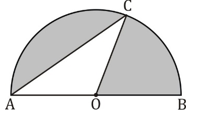(A) Length of AO is given.
(B) AC is given in multiple of radius of semicircle.

(a) Only A
(b) Only B
(c) Either Only A or Only B
(d) A and B together
(e) A and B together are not sufficient

Q2. In a box three type of balls are there, Black, Red and White. If no. of white balls is given then find out the probability of getting one white ball.
(A) Probability of getting one Red ball is given.
(B) Probability of getting one black ball is given.

(a) Only A
(b) Only B
(c) Either Only A or Only B
(d) A and B together
(e) A and B together are not sufficient

Q3. What is the volume of the sphere?
(A) Surface area of hemisphere is equal to the total surface area of the cylinder having radius and height in ratio 3 : 4.
(B) When we cut sphere into two hemi-sphere then total surface area is equal to the area of a circle whose radius is 21 cm.

(a) Only A
(b) Only B
(c) Either Only A or Only B
(d) A and B together
(e) A and B together are not sufficient

Q4. PR is diameter of circle. Find, ∠QPO – ∠SRO = ?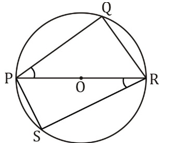(A) ∠SPO = 40°
(B) ∠PQR + ∠QRO = 120°

(a) Only A
(b) Only B
(c) Either Only A or Only B
(d) A and B together
(e) A and B together are not sufficient

Q5. A shopkeeper gets a loss of 70 Rs. when he sold an article at 20% discount on M.P. Find cost price of Article.
(A) % of mark up above cost price is equal to % discount given on M.P.
(B) when no discount is given, article sold at profit of 350 Rs.

(a) Only A
(b) Only B
(c) Either Only A or Only B
(d) A and B together
(e) A and B together are not sufficient

Directions (6-10): The following questions are accompanied by two statements A and B. You have to determine which statements(s) is/are sufficient/necessary to answer the questions.
(a) Statement A alone is sufficient to answer the question but statement B alone is not sufficient to answer the questions.
(b) Statement B alone is sufficient to answer the question but statement A alone is not sufficient to answer the question.
(c) Both the statements taken together are necessary to answer the questions, but neither of the statements alone is sufficient to answer the question.
(d) Either statement A or statement B by itself is sufficient to answer the question.
(e) Statements A and B taken together are not sufficient to answer the question.

Q6. An equilateral triangle is inscribed in a circle. What will be the difference between the area of circle and area of triangle?
(a) Radius of circle is given
(b) Sum of the perimeter of triangle and circle is given.

Q7. Is ‘n’ even or odd, if n is a natural number.
(a) 2n + 1 is divisible by 3
(b) 4n – 1 is divisible by 3

Q8. Five positive naturals numbers are given which may or may not be equal the sum of these numbers.
(a) If numbers are arranged is ascending order then median is 18 while sum of first and last number and second and fourth number is equal
(b) Median of these number is 18 while mode of these numbers is 12 and 24.

Q9. ‘X’ mark up an article 50% above its cost price. Find the cost price of article.
(a) ‘X’ gave Rs 60 discount on mark price and earn 20% profit.
(b) If ‘X’ gave two successive discounts of 10% each then he will earn Rs 43 as profit.

Q10. Amit tells truth 2 times out of x times while Ankush tells truth 5 times out of ‘y’ times. Find the product of ‘x’ and ‘y’
(a) Probability that Amit and Ankush contradict with each other on facts is 50%
(b) Probability that both tells truth is 20%

Directions (11–15): In the given questions, two quantities are given, one as ‘Quantity I’ and another as ‘Quantity II’. You have to determine relationship between two quantities and choose the appropriate option

Q11. Total surface area of cube and sphere are equal.
Quantity I: Volume of cube ÷ √π
Quantity II: volume of sphere
(a) Quantity I > Quantity II
(b) Quantity I < Quantity II
(c) Quantity I ≥ Quantity II
(d) Quantity I ≤ Quantity II
(e) Quantity I = Quantity II or No relation

Q12. A dice is thrown. If an even number comes, then a coin is tossed once but if an odd prime number comes, then two coins are tossed.
Quantity I: Probability of getting exactly one head.
Quantity II : Probability of getting at least one tail.
(a) Quantity I > Quantity II
(b) Quantity I < Quantity II
(c) Quantity I ≥ Quantity II
(d) Quantity I ≤ Quantity II
(e) Quantity I = Quantity II or No relation

Q13. Figure I and II shows two semicircles of radius 14 cm with O and P as their centres respectively.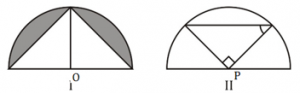Quantity I: Area of shaded region in figure I
Quantity II: Area of triangle in figure II
(a) Quantity I > Quantity II
(b) Quantity I < Quantity II
(c) Quantity I ≥ Quantity II
(d) Quantity I ≤ Quantity II
(e) Quantity I = Quantity II or No relation

Q14. When a 30% discount is given on marked price of an article, 20% profit is earned . If article is sold for Rs 35 more, then value of profit percent increases by 50%.
Quantity I: Selling price of article after Rs 35 more is added.
Quantity II: Selling price of article when it sold at 24⅙% discount.
(a) Quantity I > Quantity II
(b) Quantity I < Quantity II
(c) Quantity I ≥ Quantity II
(d) Quantity I ≤ Quantity II
(e) Quantity I = Quantity II or No relation

Q15. Number of ways in which word ‘SOMETHING’ can be arranged.
Quantity I : When all vowels comes together.
Quantity II: When all consonants comes together.
(a) Quantity I > Quantity II
(b) Quantity I < Quantity II
(c) Quantity I ≥ Quantity II
(d) Quantity I ≤ Quantity II
(e) Quantity I = Quantity II or No relation

Solutions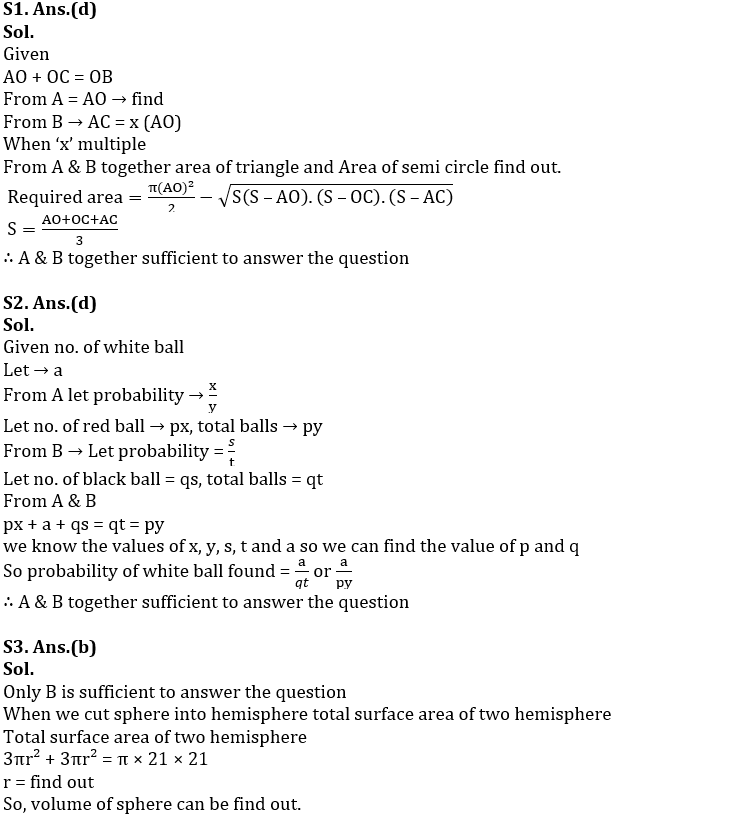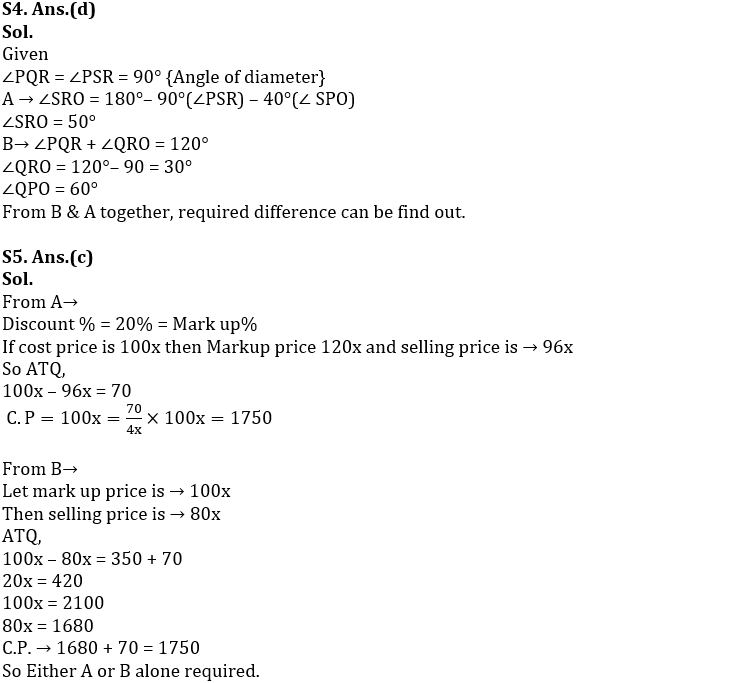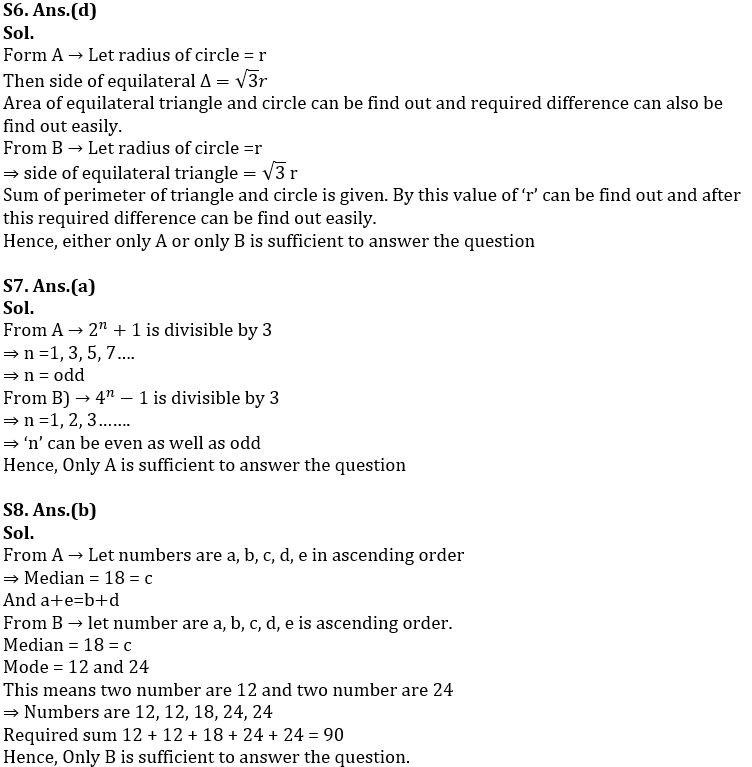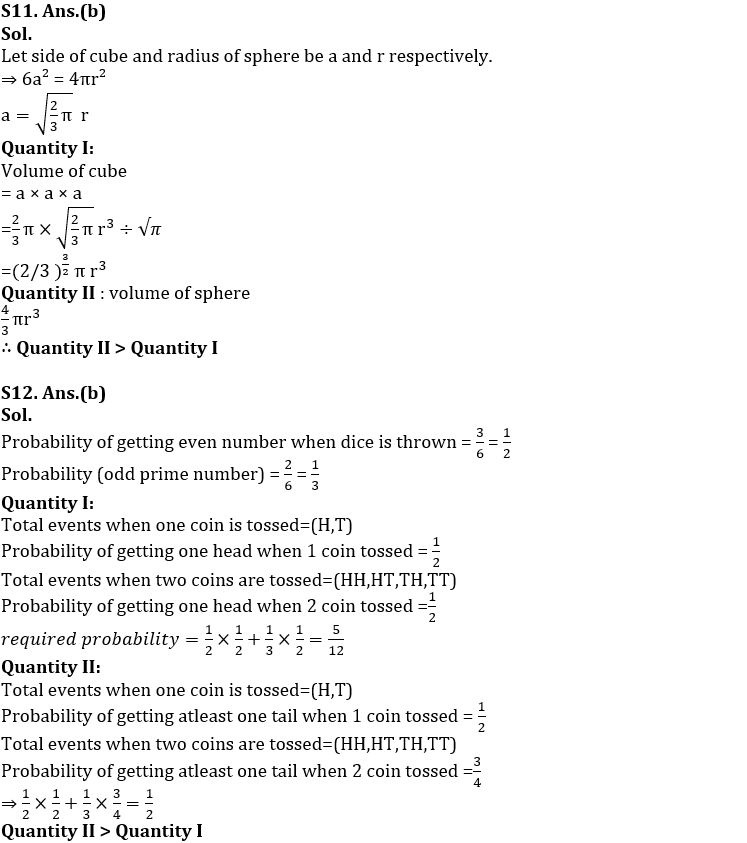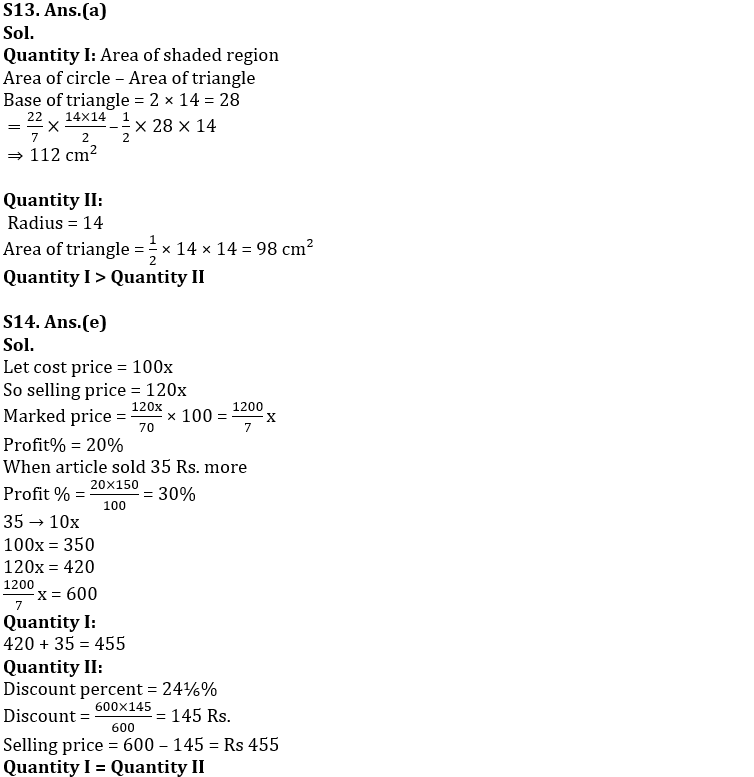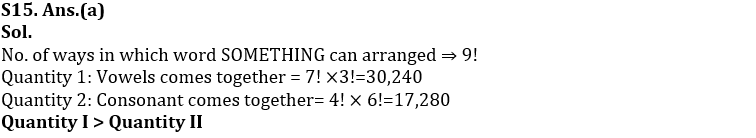#### Congratulations!